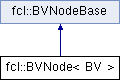FCL  0.6.0 Flexible Collision Library
fcl::BVNode< BV > Struct Template Reference

A class describing a bounding volume node. It includes the tree structure providing in BVNodeBase and also the geometry data provided in BV template parameter. More...

`#include <BV_node.h>`

Inheritance diagram for fcl::BVNode< BV >:## Public Types

using S = typename BV::S

## Public Member Functions

bool overlap (const BVNode &other) const
Check whether two BVNode collide.

distance (const BVNode &other, Vector3< S > *P1=nullptr, Vector3< S > *P2=nullptr) const
Compute the distance between two BVNode. P1 and P2, if not nullptr and the underlying BV supports distance, return the nearest points.

Vector3< S > getCenter () const
Access the center of the BV.

Matrix3< S > getOrientation () const
Access the orientation of the BV.Public Member Functions inherited from fcl::BVNodeBase
bool isLeaf () const
Whether current node is a leaf node (i.e. contains a primitive index.

int primitiveId () const
Return the primitive index. The index is referred to the original data (i.e. vertices or tri_indices) in BVHModel.

int leftChild () const
Return the index of the first child. The index is referred to the bounding volume array (i.e. bvs) in BVHModel.

int rightChild () const
Return the index of the second child. The index is referred to the bounding volume array (i.e. bvs) in BVHModel.

## Public Attributes

BV bv
bounding volume storing the geometryPublic Attributes inherited from fcl::BVNodeBase
int first_child
An index for first child node or primitive If the value is positive, it is the index of the first child bv node If the value is negative, it is -(primitive index + 1) Zero is not used.

int first_primitive
The start id the primitive belonging to the current node. The index is referred to the primitive_indices in BVHModel and from that we can obtain the primitive's index in original data indirectly.

int num_primitives
The number of primitives belonging to the current node.

## Detailed Description

### template<typename BV> struct fcl::BVNode< BV >

A class describing a bounding volume node. It includes the tree structure providing in BVNodeBase and also the geometry data provided in BV template parameter.

The documentation for this struct was generated from the following files:
• /home/js/dev/fcl/master/include/fcl/geometry/bvh/BV_node.h
• /home/js/dev/fcl/master/include/fcl/geometry/bvh/BV_node-inl.h